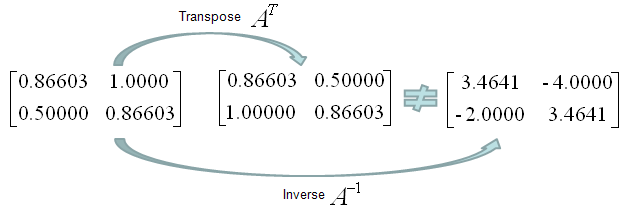Engineering Math - Quick Reference                                 Home : www.sharetechnote.com Matrix-Orthogonal Matrix   In simple description, the Orthogonal Matrix is a special form of matrix in which each of the column vectors or row vectors are orthogonal to each other. (You may ask what is 'Orthogonal' ? The, refer to Orthogonality Page).   If I list up the properties of Orthogonal Matrix in more details, it is as follows It is a Square Matrix Each of the column vectors or row vectors are orthogonal to each other Following equation are always met (Inner product of a Matrix and its transpose produce an Identity Matrix)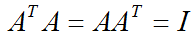Following equation are always met (Transpose of the matrix is same as the inverse of the matrix.)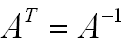A couple of examples would give you better understanding. Is following matrix (the matrix in the left). Yes it is because its transpose and inverse are same.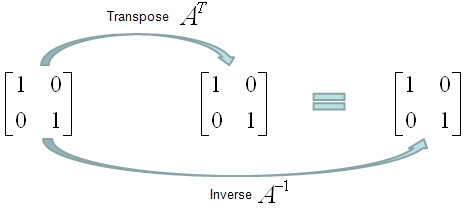Is following matrix (the matrix in the left) orthogonal. Yes it is because its transpose and inverse are same.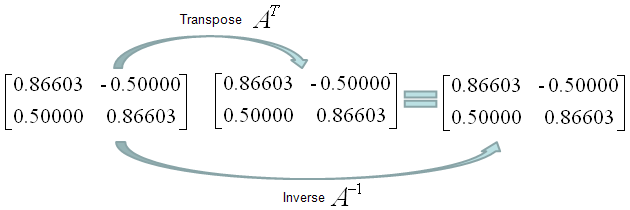Is following matrix (the matrix in the left) orthogonal. No it is not because its transpose and inverse are not same.